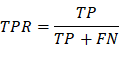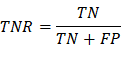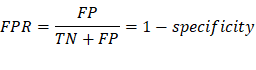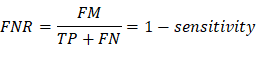Confusion Matrix

Example Codes:

A confusion matrix, also known as an error matrix, is a specific table layout that allows visualization of the performance of an algorithm. It is a special kind of contingency table, with two dimensions ("actual" and "predicted"), and identical sets of "classes" in both dimensions (each combination of dimension and class is a variable in the contingency table).

The Layout of the confusion matrix is as follow:

 Predicted Condition Total population = P + N Positive (PP) Negative (PN) Actual condition Positive (P) True Positive (TP) False Negative (FN) Negative (N) False Positive (FP) True Negative (TN)

1.     True Positive (TP): A test result that correctly indicates the presence of a condition or characteristic.

2.     True Negative (TN): A test result that correctly indicates the absence of a condition or characteristic.

3.     False Positive (FP): A test result which wrongly indicates that a particular condition or attribute is present.

4.     False Negative (FN): A test result which wrongly indicates that a particular condition or attribute is absent.

Terms Used

TPR/Sensitivity: True positive rate, measures the proportion of positives that are correctly identified(correctly identify those with a disease).TNR/Specificity: True negative rate, measures the proportion of negatives

that are correctly identified (correctly identify those without a disease).FPR/Type I Error: False positive rate, measures the proportion of negatives that are wrongly categorized as positives. (The probability of rejecting the null hypothesis when it’s true)FNR/Type II Error: False negative rate, measures the proportion of positives that are wrongly categorized as negatives. (The probability of accepting the null hypothesis when it’s false)R Example

#Install required packages

install.packages('caret')

#Import required library

library(caret)

#Creates vectors having data points

expected_value <- factor(c(1,0,1,0,1,1,1,0,0,1))

predicted_value <- factor(c(1,0,0,1,1,1,0,0,0,1))

#Creating confusion matrix

example <- confusionMatrix(data=predicted_value, reference = expected_value)

#Display results

example

#Simpler way

table(expected_value,predicted_value)

SAS Example

data predicts;

input expect predict;

datalines;

1 1

0 0

1 0

0 1

1 1

1 1

1 0

0 0

0 0

1 1

;

proc freq data=predicts;

tables expect*predict;

run;